# Optimal control, mathematical theory of

Jump to: navigation, search

A part of mathematics in which a study is made of ways of formalizing and solving problems of choosing the best way, in an a priori described sense, of realizing a controlled dynamical process. This dynamical process, as a rule, can be described using differential, integral, functional, and finite-difference equations (or other formalized evolution relations, possibly involving stochastic aspects), depending on input functions or parameters, called controls, and usually subject to constraints. The sought controls, as well as the realization of the process itself, must generally be chosen according to certain constraints prescribed by the formulation of the problem.

In a more specific sense, it is accepted that the term "mathematical theory of optimal control" be applied to a mathematical theory in which methods are studied for solving non-classical variational problems of optimal control (as a rule, with differential constraints), which permit the examination of non-smooth functionals and arbitrary constraints on the control parameters or on other dependent variables (the constraints which are usually studied are given by non-strict inequalities). The term "mathematical theory of optimal control" is sometimes given a broader meaning, covering the theory which studies mathematical methods of investigating problems whose solutions include any process of statistical or dynamical optimization, while the corresponding model situations permit an interpretation in terms of some applied procedure for adopting an optimal solution. With this interpretation, the mathematical theory of optimal control contains elements of operations research; mathematical programming and game theory (cf. Games, theory of).

Problems studied in the mathematical theory of optimal control have arisen from practical demands, especially in space flight dynamics and automatic control theory (see also Variational calculus). The formalization and solution of these problems have posed new questions, for example in the theory of ordinary differential equations, both in the area of generalizing the concept of a solution and generalizing conclusions from the appropriate conditions of existence, as in the study of dynamical and extremal properties of trajectories of controlled differential systems. In particular, the mathematical theory of optimal control has stimulated the study of the properties of differential inclusions (cf. Differential inclusion). The corresponding directions in the mathematical theory of optimal control are therefore often considered as part of the theory of ordinary differential equations. The mathematical theory of optimal control contains the mathematical basis of the theory of controlled motions, a new area in general mechanics in which the laws of creating controllable mechanical movements and related mathematical questions are investigated. In methods and in applications, the mathematical theory of optimal control is closely linked with analytical mechanics, especially with the areas relating to the variational principles of classical mechanics.

Although particular problems of optimal control and non-classical variational problems were encountered earlier, the foundations of the general mathematical theory of optimal control were laid in 1956–1961. The key point of this theory was the Pontryagin maximum principle, formulated by L.S. Pontryagin in 1956 (see ). The main stimuli in the creation of the mathematical theory of optimal control were the discovery of the method of dynamic programming, the explanation of the role of functional analysis in the theory of optimal systems, the discovery of connections between solutions of problems of optimal control and results of the theory of Lyapunov stability and the appearance of works relating to the concepts of controllability and observability of dynamical systems (see ). In the ensuing years the foundations have been laid of the theory of stochastic control and stochastic filtering of dynamical systems, general methods have been created for the solution of non-classical variational problems, generalizations of the basic statements of the mathematical theory of optimal control have been obtained for more complex classes of dynamical systems, and the connections with classical variational calculus have been studied (see ). The mathematical theory of optimal control is developing intensively, especially in the study of game problems in dynamics (see Differential games), problems of control with incomplete or imprecise information, systems with distributed parameters, equations on manifolds, etc.

The results of the mathematical theory of optimal control have found broad applications in the construction of control processes relating to diverse areas of modern technology, in the study of economic dynamics, and in the solution of a number of problems in the fields of biology, medicine, ecology, demography, etc.

A problem of optimal control can be described in general terms as follows:

1) A controllable systemis given, whose position at an instant of timeis represented by a value(for example, by a vector of generalized coordinates and impulses of a mechanical system, or by a function in the spatial coordinates of a distributed system; by a probability distribution which characterizes the current state of a stochastic system, or by a vector of production output in a dynamic model of economy, etc.). It is assumed that a number of controlsmay be applied to the systemaffecting the dynamics of the system. The controls might take the form of mechanical forces, thermal or electrical potentials, investment programs, etc.

2) An equation is given which connects the variables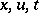and describes the dynamics of the system. An instant of time is indicated at which the equation is considered. In a typical case one considers an ordinary differential equation of the form(1)

with previously stipulated properties of the function(continuity ofin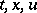and continuous differentiability inare often required).

3) Information is available which can be used to construct the controls (for example, at any instant of time or at previously prescribed instances, the allowed measurable values of the phase coordinates of the system (1) or of functions in these coordinates become known). A class of functions describing the controls which can be considered is stipulated: The set of piecewise-continuous functions of the form, the set of linear functions inof the form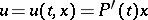with continuous coefficients, etc.

4) Constraints are imposed on the process to be realized. At this point in particular, the conditions defining the aim of the control come into consideration (for example, for the system (1), to hit a given point or a given set of the phase space, the demand for stabilization of the solutions around a given motion, etc.). Furthermore, constraints can be imposed on the values of the controlsor on the coordinates of the position, on functions in these variables, on functionals in their realizations, etc. In the system (1), for example, constraints on the control parameters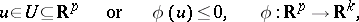(2)

and on the coordinates(3)

are possible; here,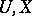are closed sets and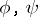are differentiable functions. More complex situations can also be examined, where the setdepends on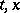or when an inequality in the form(a case of mixed constraints) is given, etc.

5) An index (a criterion) is given of the quality of the process to be realized. It can take the form of a functional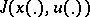in the realization of the variablesover the period of time under consideration. Conditions 1)–4) are now supplemented by the requirement of optimality of the process: a minimum, maximum, minimax, etc., of the index.

In this way, in a given class of controls for a given system, a controlmust be chosen which optimizes (i.e. minimizes or maximizes) the index(under the condition that the aim of the control is achieved and that the applied constraints are fulfilled). A function (e.g. of the form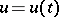or, etc.) which solves the problem of optimal control is called an optimal control (for an example of a typical statement of an optimal control problem see Pontryagin maximum principle).

Among the dynamic objects embraced by the problems of the mathematical theory of optimal control, it is customary to differentiate between the finite-dimensional and the infinite-dimensional ones, dependent on the dimension of the phase space of the corresponding systems of differential equations describing them, or on the form of the constraints imposed on the phase variables.

There is a difference between problems of optimal programming control and of optimal synthesis control. In the former, the controltakes the form of a function of time; in the latter, it takes the form of a control strategy according to the feedback principle, as a function from the permissible values of the current parameters of the process. Optimal stochastic control problems are of the second type.

The mathematical theory of optimal control is concerned with questions of the existence of solutions, the derivation of necessary conditions for an extremum (optimality of a control), research into sufficient conditions, and the construction of numerical algorithms. The relations between solutions of problems of the mathematical theory of optimal control obtained in the class of programming and synthesis controls are also studied.

The different formulations of optimal control problems described above assume the existence of a correct mathematical model of the process and are calculated on the basis of complete a priori or even complete current information about the corresponding system. However, in applied formulations the accessible information on the system (for example, information about initial and final conditions, about coefficients in the corresponding equations, about the values of supplementary parameters of permissible measurable coordinates, etc.) is frequently insufficient for the direct use of the above theory. This leads to optimal control of problems formulated in other terms of information. A large section of the mathematical theory of optimal control is dedicated to problems where the description of insufficient quantities has a statistical character (the so-called theory of stochastic optimal control). If any statistical information on insufficient quantities is lacking, but only the areas in which they may alter are given, then the corresponding problems are examined within the framework of the theory of optimal control under conditions of uncertainty. Minimax and game-theoretical methods are then used to solve these problems. Problems of optimal stochastic control and of optimal control under conditions of uncertainty are especially interesting in the area of optimal synthesis control.

Although the formalized description of controllable systems can take a fairly abstract form (see ), the simplest classification also allows them to be divided into continuous-time systems (described, for example, by differential equations, either ordinary or partial, by equations with a deviating argument, by equations in a Banach space, as well as by differential inclusions, integral and integro-differential equations, etc.) and multi-stage (discrete) systems, described by recurrence difference equations and examined only at isolated (discrete) moments of time.

Discrete control systems, apart from being interesting in themselves, have great significance as finite-difference models of continuous systems. This is important for the construction of numerical methods for solving problems of optimal control (see , ), especially in those cases where the initial problem is subject to discretization, beginning with its own state. The basic formulations of the tasks shown above are also used for discrete systems. Although the functional-theoretic side of the research proves to be simpler here, a transfer of basic facts of the theory of optimal control for continuous systems and their presentation in compact form entails particular difficulties and is not always possible (see , ).

The theory of optimal linear discrete control systems with constraints defined by convex functions has been dealt with in a reasonably complete way (see ). It goes hand in hand with methods of linear and convex programming (especially with corresponding "dynamic" or "non-stationary" variants, see ). In this theory great importance is attached to solutions which allow the optimization of a discrete dynamical system to lead to the realization of an adequate numerical discrete algorithm.

Another class of problems in the mathematical theory of optimal control is generated by questions of approximation of solutions of problems of optimal control for continuous systems by discrete ones and by questions which are closely connected with the problem of regularizing ill-stated problems (see ).